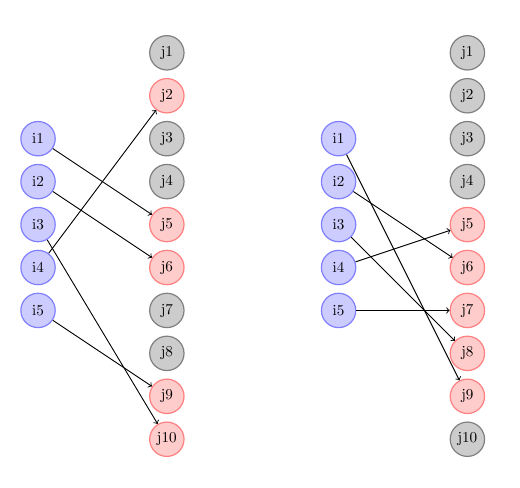## Wednesday, January 3, 2018

### Variant of an Assignment Problem

The standard assignment problem can be stated as:

\bbox[lightcyan,10px,border:3px solid darkblue]{\begin{align}\min\>&\sum_{i,j} c_{i,j} x_{i,j}\\ & \sum_j x_{i,j} = 1 && \forall i\\ & \sum_i x_{i,j} = 1 && \forall j\\&x_{i,j} \in \{0,1\} \end{align}}

There are highly efficient specialized algorithms for this problem . I often solve these problems just as an LP (the integer variables are automatically integer valued for this particular problem). That sounds like a dumb approach, but there are some reasons:

• LP solvers are actually very good in solving this quickly (often faster than a specialized method that is not implemented with very much care for efficiency ).
• Variants and extensions are (often) easily handled.

The standard model above assumes the sets $$i \in I$$ (sources) and $$j \in J$$ (destinations) have the same size. Let $$n=|I|$$ and $$m=|J|$$ be the number of $$i$$'s and $$j$$'s. Thus the standard model has $$n=m$$. Now assume we have more $$j$$'s than $$i$$'s: $$m>n$$. The model can be updated to:

\bbox[lightcyan,10px,border:3px solid darkblue]{\begin{align}\min\>&\sum_{i,j} c_{i,j} x_{i,j}\\ & \sum_j x_{i,j} = 1 && \forall i\\ & \sum_i x_{i,j} \le 1 && \forall j\\&x_{i,j} \in \{0,1\} \end{align}}

I.e. each $$i$$ is connected to a unique $$j$$, but some $$j$$'s are not assigned. A solution for a random data set with $$n=5$$ and $$m=10$$ can look like:

----     41 PARAMETER c  cost coefficients

j1          j2          j3          j4          j5          j6          j7          j8          j9         j10

i1       0.661       0.756       0.627       0.284       0.086       0.103       0.641       0.545       0.032       0.792
i2       0.073       0.176       0.526       0.750       0.178       0.034       0.585       0.621       0.389       0.359
i3       0.243       0.246       0.131       0.933       0.380       0.783       0.300       0.125       0.749       0.069
i4       0.202       0.005       0.270       0.500       0.151       0.174       0.331       0.317       0.322       0.964
i5       0.994       0.370       0.373       0.772       0.397       0.913       0.120       0.735       0.055       0.576

----     41 VARIABLE x.L  assignment variables

j2          j5          j6          j9         j10

i1                       1
i2                                   1
i3                                                           1
i4           1
i5                                               1


Let's add a picture to this post (created with tikz):Original assignment and new variant

In  an unusual variant is asked for: the assigned $$j$$'s should be adjacent. The required configuration can be seen in the right picture above.

The first thing we do is to keep track if a $$j$$ node is assigned. For this we introduce new binary variables $$y_j$$ and replace the second assignment constraint $$\sum_i x_{i,j} \le 1$$ by:

\begin{align} &y_j = \sum_i x_{i,j}\\&y_j \in \{0,1\}\end{align}

The variable $$y_j$$ will be zero for the unassigned nodes (grey in the picture above), and one if connected (red). We can make sure we don't have "holes" in the $$y$$ vector by requiring that we see the pattern $$[0,\> 1]$$  only once. This we can model as:

\begin{align} &z_j \ge y_j - y_{j-1}\\& \sum_j z_j \le 1\\&z_j \in \{0,1\} \end{align}

This approach is often used in machine scheduling or power generation: prevent a generator to be turned on too many times.

This model has as results:

----     44 VARIABLE x.L  assignment variables

j5          j6          j7          j8          j9

i1                                                           1
i2                       1
i3                                               1
i4           1
i5                                   1

----     44 VARIABLE y.L  destination is used

j5 1,    j6 1,    j7 1,    j8 1,    j9 1

----     44 VARIABLE z.L  0-1 transition

j5 1


The complete GAMS model looks like:

Notes:

• The variables $$y$$ and $$z$$ can be relaxed to be continuous between zero and one.
• The $$x$$ variables are no longer automatically integer valued. We need to solve this model as a real MIP and can not use just an LP solver.
• When using leads and lags we always have to look at the boundary case. Here we have the special case $$z_1 \ge y_1 - 0$$. In GAMS when we index out-of-domain we get a zero. That is exactly what we need in this case.
• The problem can also be attacked by solving $$m-n+1$$ assignment problems (and then pick the best one).

#### References

1. Rainer Burkard,‎ Mauro Dell'Amico,‎ Silvano Martello, Assignment Problems, SIAM, 2009
2. Assignment problem, http://yetanothermathprogrammingconsultant.blogspot.com/2009/11/assignment-problem.html
3. Combinatorial optimization: assignment (matching) with “consecutive” assignments, https://stackoverflow.com/questions/48049506/combinatorial-optimization-assignment-matching-with-consecutive-assignments